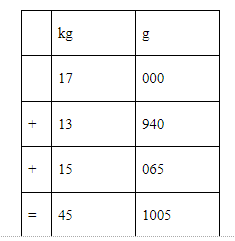QuestionAnswers

# Add 17 kg, 13 kg 940 g and 15 kg 65 gA. 40 kg 65 gB. 4 kg 650 gC. 46 kg 50 gD. 46 kg 5 g

Hint: Mass is commonly measured by how much something weighs. In the metric system of measurement, the most common units of mass are the gram and kilogram.
Rules of addition/ subtraction are as follows:
1. Write the numbers to be added/subtracted in vertical form.
2. While adding/subtracting units write them in separate columns, i.e., ‘kg’ and ‘g’ to be written in
separate columns.
To convert kilograms into grams, multiply the given measure in kilograms by 1000 and write the unit gram (g) after it.
$1kg = 1000g$
To convert the grams into kilograms and grams, the last 3 digits from the right represent the number of grams and the rest of the digits represent the number of kilograms. For eg:
7216 g = 7 kg 216 g

Complete step by step solution: We have to add the terms 17 kg, 13 kg 940 g and 15 kg 65 g
We will arrange all the terms into column and add themTherefore, the sum is 45 kg 1005 g
Now, $1kg = 1000 g$ so we can convert 1005 g into kg
We can write 1005 g as
$\begin{gathered} 1005g = 1000g + 005g \\ 1005g = 1kg + 5g \\ \therefore 1005g = 1kg5g \\ \end{gathered}$
Therefore, 45 kg 1005 g will be equal to 46 kg 5 g
Hence, the sum is 46 kg 5 g
∴Option (D) is correct

Note: 1. To convert kilograms to grams we have to multiply the number of kilograms by 1000 and to convert grams to kilograms we divide the number of grams by 1000.